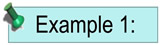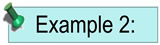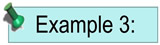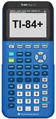Solving Linear Quadratic Systems Algebraically MathBitsNotebook.com Terms of Use   Contact Person: Donna RobertsAs a reference see: "What Do Linear Quadratic Systems Look Like?"

A linear quadratic system is a system containing one linear equation and one quadratic equation
which may be one straight line and one parabola,
or one straight line and one circle.Algebraic Solutions
straight line:   y = mx + b
parabola:   y = ax2 + bx + c;   a 0
circle:   (x - h)2 + (y - k)2 = r2 ; center (h.k), radius ry = x2- 6x + 3 (parabola)
y = -2x + 3 (straight line)
1. Solve for one of the variables in the linear equation.
Note: In this example, this process is already done for us, since y = -2x + 3.
y = -2x + 3
2. Substitute this value into the quadratic equation, and solve the resulting equation.

• Substitute -2x + 3 for y in the quadratic equation.
• Subtract 3 from both sides; then add 2x to both sides.
• Factor.
• Set each factor equal to zero and solve.

You now have TWO values for x. This tells you that there may be two possible solutions.
TWO SOLUTIONS.
y = x2 - 6x + 3
-2x + 3 = x2 - 6x + 3
0 = x2 - 4x + 0
0 = x(x - 4)

x = 0;    x - 4 = 0

x = 0;     x = 4

3. Find the corresponding values for y. Substitute each value into the linear equation in place of x.

Yes, you could substitute in the quadratic equation, but substituting into the linear equation will be easier.

x = 0
y
= -2(
0) + 3
y = 3
Solution 1:
x = 0; y = 3
or (0, 3)
x = 4
y = -2(4) + 3
y = -5
Solution 2:
x = 4; y = -5
or (4, -5)
4. Check: Be sure to check BOTH solutions in both equations.
 Solution 1: x = 0; y = 3 y = -2x + 3 3 = -2(0) + 3 3 = 3 check! y = x2 - 6x + 3 3 = (0)2 - 6(0) + 3 3 = 3 check! Solution 2: x = 4; y = -5 y = -2x + 3 -5 = -2(4) + 3 -5 = -5 check! y = x2 - 6x + 3 -5 = (4)2 - 6(4) + 3 -5 = 16 - 24 + 3 -5 = -5 check!5. State the final solutions. The solutions may be stated as the set {(0, 3), (4, -5)}y = x2- 6x + 3 (parabola)
2x - y = 13 (straight line)
1. Solve for one of the variables in the linear equation.
2x - y = 13
y = 2x - 13
2. Substitute this value into the quadratic equation, and solve the resulting equation.

• Substitute 2x - 13 for y in the quadratic equation.
• Add 13 to both sides; then subtract 2x from both sides.
• Factor.
• Set each factor equal to zero and solve.

You now have ONE value for x. This tells you that there may be only one solution.
ONE SOLUTION
y = x2 - 6x + 3
2x - 13 = x2 - 6x + 3
0 = x2 - 8x + 16
0 = (x - 4)(x - 4)

x - 4 = 0;    x - 4 = 0

x = 4;         x = 4
3. Find the corresponding value for y. Substitute the value into the linear equation in place of x.
Yes, you could substitute in the quadratic equation, but substituting into the linear equation will be easier.
x = 4
y
= 2(4) - 13

y = -5
Solution 1:
x = 4; y = -5
or (4, -5)
4. Check: Be sure to check the solution in both equations.
 Solution 1: x = 4; y = -5 y = 2x - 13 -5 = 2(4) - 13 -5 = -5 check! y = x2 - 6x + 3 -5 = (4)2 - 6(4) + 3 -5 = 16 - 24 + 3 -5 = -5 check!5. State the final solution. The solution may be stated as (4, -5) or {(4, -5)}x2+ y2 = 9 (circle)
x - y = 3 (straight line)
1. Solve for one of the variables in the linear equation.
y = x - 3
2. Substitute this value into the quadratic equation, and solve the resulting equation.

• Substitute x - 3 for y in the quadratic equation.
• Expand (x - 3)2
• Combine terms.
• Factor.
• Set each factor equal to zero and solve.

You now have TWO values for x. This tells you that there may be two possible solutions.
TWO SOLUTIONS.
x2 + (x - 3)2 = 9
x2 + x2 - 6x + 9 = 9
2x2 - 6x = 0
2x(x - 3) = 0

2x = 0;    x - 3 = 0

x = 0;     x = 3
3. Find the corresponding values for y. Substitute each value into the linear equation in place of x.

Yes, you could substitute in the quadratic equation, but substituting into the linear equation will be easier.

x = 0
y
= 0 - 3
y = -3
Solution 1:
x = 0; y = -3
or (0, -3)
x = 3
y = 3 - 3
y = 0
Solution 2:
x = 3; y = 0
or (3, 0)
4. Check: Be sure to check BOTH solutions in both equations.
 Solution 1: x = 0; y = -3 y = x - 3 -3 = 0 - 3 -3 = -3 check! x2 + y2 = 9 02 + (-3)2 = 9 9 = 9 check! Solution 2: x = 3; y = 0 y = x - 3 0 = 3 - 3 0 = 0 check! x2 + y2 = 9 32 + 02 = 9 9 + 0 = 9 9 = 9 check!5. State the final solutions. The solutions may be stated as the set {(0, -3), (3, 0)}Yes, this is an algebraic solution method. But, you can still use your graphing calculator to double CHECK your solution. Click the calculator at the right to see how to use the calculator with linear quadratic systems.For calculator help with linear quadratic systems click here.